# Physics - Electromagnetism - Electric potential energySource

## Introduction

Hello it's a me again Drifter Programming!

Today we continue with Electromagnetism to get into Electric potential energy!

A lot is based on Work and Energy of Classical Mechanics that I didn't do yet there, but I will cover any part needed for sure!

So, without further do, let's dive straight into it!

## Quick explanation of Work and Energy

When a Force F acts on an object that moves from a point a to a point b, then the Work W that is produced by that Force is: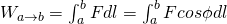quicklatex

where dl are small distances along the trajectory of the object and φ is the angle between F and dl at each point of the trajectory.

Work and Energy are calculated in Joule's (J).

When the Forces of a Force-Field are conservative then the Work can always be expressed using some potential energy U.

So, when the potential energy is Ua at some point and Ub at some other point then: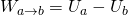quicklatex

The Work is positive when Ua is greater then Ub, which means that the potential energy decreases.

Always remember that the Force produces positive Work when the potential energy decreases!

A nice example of Classical mechanics is throwing a ball:

• When the ball is going down then gravity produces a positive work, while the potential energy decreases!
• When the ball is thrown vertically upwards then the work of gravity is negative and the potential energy increases!

The work-energy theorem tells us that the change of kinetic energy (Ka - Kb) is equal to the total work applied to an object.

The total energy at any point is constistent and so: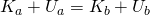quicklatex

where K is the kinetic energy and U the potential energy at points a and b.

Let's get all that into Electromagnetism now!

## Getting into Electric Potential energy

Suppose we have two parallel oppositely charged metal plates (+ on top) that produce an uniform electric field E. The electric field of course applies a force F downwards to any positive test charge q', whenever it moves a distance d from a point A to a point B.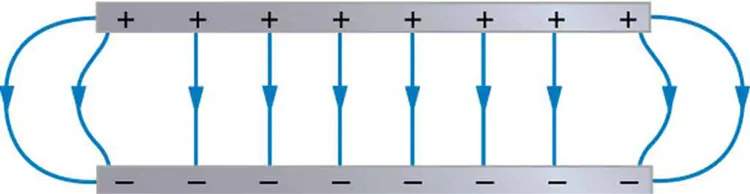Source

The force is of course independent of the location of the test charge and also consistent and so the Work produced by the electric field E is: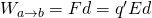quicklatex

This Work can also be expressed using a potential energy U.

The y-component of the electric force is Fy = -q'E and consistent and there also are no x and z components. This means that the Work produced is independent of the trajectory that the charge takes from a point a to a point b, but only depends on the start and endpoint.

The same way as potential energy of gravity Fy = -mg is U = mgy, the potential energy of the force of an electric field Fy = -q'E is:

U = q'Ey

And so when a test charge moves from a height ya to a height yb, the Work produced on that charge from the field is given by:quicklatex

When the height ya is greater then yb then the charge moves in the direction of the electric field E, the potential energy U is drecreasing and the Electric field produces a positive Work.

In the same way, when ya is smaller then yb then the charges moves oppositely to E, the potential energy U increases and the field produces a negative Work.

More specifically if we set ya = d and yb = 0 then: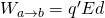quicklatex

Until now we only had a positive charge...

What's true for negative charges?

When a test charge q' is negative then the force applied goes in the opposite direction of the field and so:

• The potential energy increases when the charge moves in the direction of the field and decreases when the charge goes in the opposite direction.
• The work of the field is negative when the charge moves in the direction of the field and positive when the charge moves in the opposite direction (and so the direction of the force applied to it!)

## Electric Potential Energy

Let's now generalize it and talk about the Work that is produced on a test charge q', when it moves in the electric field of a static point charge q.

Let's first get into a trajectory along the radial line from a point a to b.

The radial component of the force using Coulomb's law is of course:
quicklatex

When q and q' are oppositely charged then the Force is negative (attract) and when the charge have the same sign then the force is positive (repel).

The force is not the same at a and b, because the distance r changes and so we need integration!

The Work that is produced to q' from the force Fr (that is applied by a charge q), while q' moves from a point a to a point b is:quicklatex

We can clearly see that the Work at that trajectory depends only on the start and end points!

In reality, the work is the same for any possible trajectory from point a to b.

Proof?

Let's suppose two points a and b that are not on the same radial line.

The Work produced to q' along such a trajectory is given by: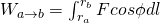quicklatex

By making a schema we would see that cosφ dl = dr. I don't found a good one... :(

Anyway, that means that the work produces at a small displacement dl depends only on the change dr of the radial distance (and so component) r between the charges.

And so, the Work produced to q' from the field E that is generated by q is independent of the trajectory and only depends on ra and rb, which are the radial distances of the start and end points!

Something interesting is that if we make a cycle and start from a point A and go to the same point A, in the end the work produced is zero!

Of course all this works only if the force-field is conservative, something that is true for electromagnetic forces!

So, by using the equations we covered 'till now we can now say that the potential energy when moving along a distance r is: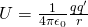quicklatex

Which of course is true for any charge q' and q!

We could also use a spherical uniform distribution of charge instead of a point charge q that produces an electric field, as we already know from Gauss's law, cause such a distribution works like it has all the charge concentrated in the center!

The potential energy depends the point of reference, where we have U = 0.

U = 0 when the distance between the charges q and q' is infinite, r = ∞.

One last thing is...what do we do when there are more charges?

Well, we again use the superposition principle...

Which means that the potential energy of a test charge q' at distances r1, r2, r3, ... away of some charges q1, q2, q3, ... is the algebraic (not vector!) sum: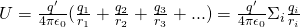quicklatex

Intro

Physics Introduction -> what is physics?, Models, Measuring

Vector Math and Operations -> Vector mathematics and operations (actually mathematical analysis, but I don't got into that before-hand :P)

Classical Mechanics

Velocity and acceleration in a rectlinear motion -> velocity, accelaration and averages of those

Rectlinear motion with constant accelaration and free falling -> const accelaration motion and free fall

Rectlinear motion with variable acceleration and velocity relativity -> integrations to calculate pos and velocity, relative velocity

Rectlinear motion exercises -> examples and tasks in rectlinear motion

Position, velocity and acceleration vectors in a plane motion -> position, velocity and accelaration in plane motion

Projectile motion as a plane motion -> missile/bullet motion as a plane motion

Smooth Circular motion -> smooth circular motion theory

Plane motion exercises -> examples and tasks in plane motions

Force and Newton's first law -> force, 1st law

Mass and Newton's second law -> mass, 2nd law

Newton's 3rd law and mass vs weight -> mass vs weight, 3rd law, friction

Applying Newton's Laws -> free-body diagram, point equilibrium and 2nd law applications

Contact forces and friction -> contact force, friction

Dynamics of Circular motion -> circular motion dynamics, applications

Object equilibrium and 2nd law application examples -> examples of object equilibrium and 2nd law applications

Contact force and friction examples -> exercises in force and friction

Circular dynamic and vertical circle motion examples -> exercises in circular dynamics

Electromagnetism

Getting into Electromagnetism -> electromagnetim, electric charge, conductors, insulators, quantization

Coulomb's law with examples -> Coulomb's law, superposition principle, Coulomb constant, how to solve problems, examples

Electric fields and field lines -> Electric fields, Solving problems around Electric fields and field lines

Electric dipoles -> Electric dipole, torque, potential and field

Electric charge and field Exercises -> examples in electric charges and fields

Electric flux and Gauss's law -> Electric flux, Gauss's law

Applications of Gauss's law (part 1) -> applying Gauss's law, Gauss applications

Applications of Gauss's law (part 2) -> more Gauss applications

Electric flux exercises -> examples in electric flux and Gauss's law

And this is actually it for today and I hope that you learned something!

Next time we will continue on with how we calculate electric potentials!

Bye!

H2
H3
H4
3 columns
2 columns
1 column